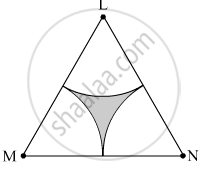SSC (Marathi Semi-English) 10thMaharashtra State Board
Share

# Δ Lmn is an Equilateral Triangle. Lm = 14 Cm. as Shown in Figure, Three Sectors Are Drawn with Vertices as Centres and Radius 7 Cm. Find, (2) Area of Any One of the Sectors . - SSC (Marathi Semi-English) 10th - Geometry

ConceptAreas of Sector and Segment of a Circle

#### Question

$∆$ LMN is an equilateral triangle. LM = 14 cm. As shown in figure, three sectors are drawn with vertices as centres and radius 7 cm.
Find,
(2) Area of any one of the sectors .#### Solution

∆LMN is an equilateral triangle.
∴ LM = MN = LN = 14 cm
∠L = ∠M = ∠N = 90º

(2) Radius of the each sector, r = 7 cm
Area of any one of the sectors =

$\frac{\theta}{360° } \times \pi r^2 = \frac{60° }{360° } \times \frac{22}{7} \times \left( 7 \right)^2$  = 25.67 cm2

Is there an error in this question or solution?

#### APPEARS IN

Solution Δ Lmn is an Equilateral Triangle. Lm = 14 Cm. as Shown in Figure, Three Sectors Are Drawn with Vertices as Centres and Radius 7 Cm. Find, (2) Area of Any One of the Sectors . Concept: Areas of Sector and Segment of a Circle.
S